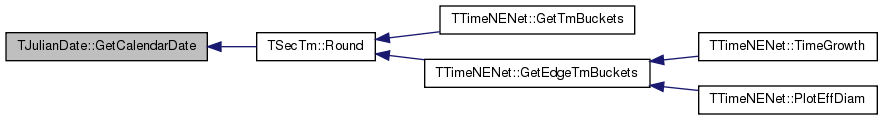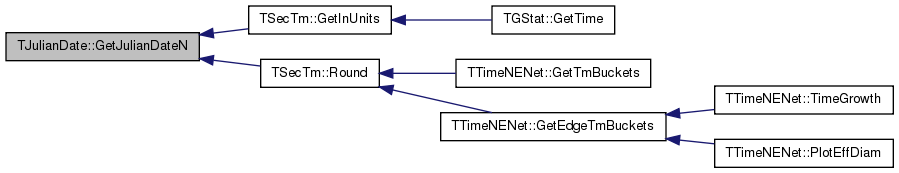SNAP Library 6.0, Developer Reference  2020-12-09 16:24:20 SNAP, a general purpose, high performance system for analysis and manipulation of large networks
TJulianDate Class Reference

`#include <tm.h>`

## Static Public Member Functions

static int GetJulianDateN (int d, int m, int y)

static void GetCalendarDate (int jdn, int &dd, int &mm, int &yy)

## Static Public Attributes

static int LastJulianDate =15821004

static int LastJulianDateN =2299160

## Detailed Description

Definition at line 69 of file tm.h.

## Member Function Documentation

 void TJulianDate::GetCalendarDate ( int jdn, int & dd, int & mm, int & yy )
static

Definition at line 327 of file tm.cpp.

References LastJulianDateN.

Referenced by TSecTm::Round().

327  {
328  int julian = -1;
329
330  long x, z, m, d, y;
331  long daysPer400Years = 146097L;
332  long fudgedDaysPer4000Years = 1460970L + 31;
333
334  if (julian < 0){ /* set Julian flag if auto set */
335  julian = (jdn <= LastJulianDateN);}
336
337  x = jdn + 68569L;
338  if (julian){
339  x+=38;
340  daysPer400Years = 146100L;
341  fudgedDaysPer4000Years = 1461000L + 1;
342  }
343  z = 4 * x / daysPer400Years;
344  x = x - (daysPer400Years * z + 3) / 4;
345  y = 4000 * (x + 1) / fudgedDaysPer4000Years;
346  x = x - 1461 * y / 4 + 31;
347  m = 80 * x / 2447;
348  d = x - 2447 * m / 80;
349  x = m / 11;
350  m = m + 2 - 12 * x;
351  y = 100 * (z - 49) + y + x;
352
353  yy = (int)y;
354  mm = (int)m;
355  dd = (int)d;
356
357  if (yy <= 0){ /* adjust BC years */
358  (yy)--;}
359 }
static int LastJulianDateN
Definition: tm.h:72

Here is the caller graph for this function:int TJulianDate::GetJulianDateN ( int d, int m, int y )
static

Definition at line 303 of file tm.cpp.

References IAssert, and LastJulianDate.

Referenced by TSecTm::GetInUnits(), and TSecTm::Round().

303  {
304  IAssert(y != 0);
305  int julian = -1;
306  long jdn;
307
308  if (julian < 0){ /* set Julian flag if auto set */
309  julian = (((y * 100L) + m) * 100 + d <= LastJulianDate);}
310
311  if (y < 0){ /* adjust BC year */
312  y++;}
313
314  if (julian){
315  jdn = 367L * y - 7 * (y + 5001L + (m - 9) / 7) / 4
316  + 275 * m / 9 + d + 1729777L;
317  } else {
318  jdn = (long)(d - 32076)
319  + 1461L * (y + 4800L + (m - 14) / 12) / 4
320  + 367 * (m - 2 - (m - 14) / 12 * 12) / 12
321  - 3 * ((y + 4900L + (m - 14) / 12) / 100) / 4
322  + 1; /* correction by rdg */
323  }
324  return (int) jdn;
325 }
#define IAssert(Cond)
Definition: bd.h:262
static int LastJulianDate
Definition: tm.h:71

Here is the caller graph for this function:## Member Data Documentation

 int TJulianDate::LastJulianDate =15821004
static

Definition at line 71 of file tm.h.

Referenced by GetJulianDateN().

 int TJulianDate::LastJulianDateN =2299160
static

Definition at line 72 of file tm.h.

Referenced by GetCalendarDate().

The documentation for this class was generated from the following files: